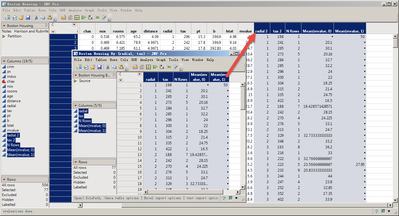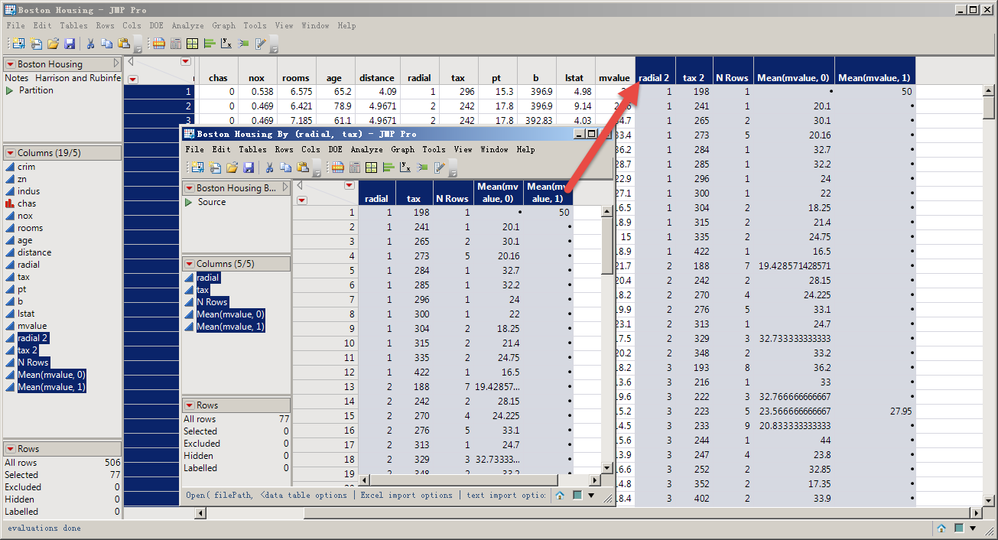Choose Language Hide Translation Bar
Highlighted

## How can I copy a new table data copy with column names to after the last column of another table?

For example, the Boston Housing.jmp Summary calculation produces a new table that wants to copy all the column names and data from the new table to the first row after the last column of Boston Housing.jmp.Thank you very much!

``````dt = Open( "\$SAMPLE_DATA/Boston Housing.jmp" );
dt << Summary(
Group( :chas, :tax ),
Mean( :mvalue ),
Freq( "None" ),
Weight( "None" )
)``````

……？？1 ACCEPTED SOLUTION

Accepted Solutions
Highlighted

## Re: How can I copy a new table data copy with column names to after the last column of another table

I'm not sure that putting summary and raw data in the same table is necessarily a good idea. But, if you need to for some reason (and if I have understood correctly), then you can use 'Tables > Join' with 'By Row Number'. In JSL:

``````Names Default To Here( 1 );

// First table
dt1 = Open( "\$SAMPLE_DATA/Boston Housing.jmp" );

// Summary table
dt2 = dt1 << Summary(
Group( :chas, :tax ),
Mean( :mvalue ),
Freq( "None" ),
Weight( "None" )
);

// Joined table
dt3 = dt1 << Join( With( dt2 ), By Row Number, Output Table( "Joined Data" ) );``````

4 REPLIES 4
Highlighted

## Re: How can I copy a new table data copy with column names to after the last column of another tableHighlighted

## Re: How can I copy a new table data copy with column names to after the last column of another table

I'm not sure that putting summary and raw data in the same table is necessarily a good idea. But, if you need to for some reason (and if I have understood correctly), then you can use 'Tables > Join' with 'By Row Number'. In JSL:

``````Names Default To Here( 1 );

// First table
dt1 = Open( "\$SAMPLE_DATA/Boston Housing.jmp" );

// Summary table
dt2 = dt1 << Summary(
Group( :chas, :tax ),
Mean( :mvalue ),
Freq( "None" ),
Weight( "None" )
);

// Joined table
dt3 = dt1 << Join( With( dt2 ), By Row Number, Output Table( "Joined Data" ) );``````

Highlighted

Highlighted

## Re: How can I copy a new table data copy with column names to after the last column of another table

Hi Ian,

this is an interesting topic. Is there away to add the summary data table to the source data table in order to have only one file (script table)?

What JSL must be applied to add the resulting means in one column and the N Rows as well in another column to the source data table?

The main interest here is to keep the summary data results in the origin source data table.

Article Labels

There are no labels assigned to this post.